# Analysis of Energy and Exergy for the Flat Plate Solar Air Collector with Longitudinal Fins Embedded in Paraffin Wax Located in Baghdad Center

Analysis of Energy and Exergy for the Flat Plate Solar Air Collector with Longitudinal Fins Embedded in Paraffin Wax Located in Baghdad Center

Abdulmunem R. Abdulmunem Mohammed Hassan JabalPakharuddin Mohd Samin Hasimah Abdul Rahman Hashim A. Hussien

School of Mechanical Engineering, Faculty of Engineering, Universiti Teknologi Malaysia, Johor 81310, Malaysia

Electromechanical Engineering Department, University of Technology, Baghdad 10066, Iraq

Center of Electrical Energy System, of Electrical Engineering, Faculty of Engineering, Universiti Teknologi Malaysia, Johor 81310, Malaysia

Corresponding Author Email:
50124@uotechnology.edu.iq
Page:
1180-1186
|
DOI:
https://doi.org/10.18280/ijht.370428
19 January 2019
|
Accepted:
4 December 2019
|
Published:
26 December 2019
| Citation

OPEN ACCESS

Abstract:

Latent heat of the fusion for the Phase changeable materials (PCM) can be utilized as heat energy store, and this energy can be employed in different applications. In present experimental work, effect of using the embedded longitudinal fins within the paraffin wax, on the energy and exergy performance for the solar air collector was investigated under the Baghdad governorate climate. Compared with the collector without paraffin wax, the results indicated that using of paraffin wax as a thermal storage material reduces the losses of energy and exergy of the collector, high levels of heat reduction by using PCM/Fins were about 33 and 40% respectively at the peak heat charging time (12:30 PM), while the decreasing in energy and exergy losses of the collector with PCM only was about 24 and 30% respectively at the same time of charging (12:30 PM). Due to the going of sum heat to store in the paraffin wax at the charging heat time, the energy along with exergy heat gains, while efficiencies were decreased with and without fins, this decreasing in the heat was the most by using PCM/Fins and it was around 30 and 44%, respectively for energy and exergy efficiencies, while it was about 20 and 31% by using PCM only at the same time of testing. The benefit of using embedded longitudinal fins in PCM is to enhance the thermal storage efficiency by about 6% compared with the solar collector that uses PCM only.

Keywords:

solar collector, PCM, fins, energy, exergy, efficiency

1. Introduction

Iraq lies in a suitable location that has received a large amount of energy due to solar radiation along the year. This energy can be used in many thermal applications such as residential, agricultural and artificial, if it utilized correctly will be very beneficial to society service. This energy is available in sunny hours of day, that led to the need for many researchers thinks’ about to store this thermal energy during the day and use it for a long time after disappearance the sun. Latent heat of fusion of the phase change of materials are one of important way can be used for thermal energy storage.

Akpinar et al.  gives an experimental investigates performance analysis of a new flat-plate solar air heater (SAH) with several obstacles and without obstacles. The outcomes of this study appeared that the efficiency of the solar air collectors depends significantly on solar radiation, the surface geometry of the collectors and the extension of the airflow line. The largest irreversibility was occurring at the SAH without obstacles (Type IV) collector in which collector efficiency is smallest. While, Abdelkader et al.  gives energy and exergy analysis of a flat-plate solar air heater coated with carbon nanotubes and cupric oxide nanoparticles embedded in black paint, the obtained outcomes display that energy efficiency enhanced by approximately 24.4%. The difference between outlet and inlet temperatures of the air across SAH rises up to 22% based on averaged values. SAH with new coating shows higher exergy efficiency. Velmurugan et al.  study (experimentally) the steady-state energy and exergy performance of single-pass flat plate solar air heater (SPFPSAH), roughened plate dual-pass solar air heater (RPDPSAH), finned plate dual-pass solar air heater (FPDPSAH) and wire mesh dual-pass solar air heater (WMDPSAH) at varied mass flow rates and solar intensities. The analytical and experimental results show that the energy and exergy performance of WMDPSAH is superior to FPDPSAH, RPDPSAH, and SPFPSAH. The pressure drop of WMDPSAH is higher than that of FPDPSAH, RPDPSAH, and SPFPSAH. Energy and exergy of a flat plate solar air heater with and without several obstacles experimentally were studied and analyzed by Esen , the results shown that using obstacles lead to improving collector efficiency, and the exergy relations are delivered for different obstacles. In a different numerical study of a water heater collector integrated with sensible and latent heat storage materials has been done by Chaabane et al. , a numerical outcome appears that during the day time, the latent heat storage material more effective, lower thermal losses and better heat preservation than the sensible heat storage material. The impact of utilizing the inner longitudinal fins in a rectangular encapsulation on the PCM (paraffin wax) melting process was studied numerically and experimentally by Abdulmunem and Jalil , the results indicated that using inner longitudinal fins led to accelerate melting of PCM (paraffin wax). While, Lin  was developed a 3D computational fluid dynamics CFD model with 6.7% and 7.1% simulation error for solar water heater, experimental results of the CFD models showed a good agreement for PCM and hot water temperatures respectively. Mehla et al.  study the performance (experimental investigation) of the evacuated tube solar air collector coupled to a latent thermal energy store for generating hot air, and the results show that this system has advantages over systems using sensible storage as it can be used after sunset due to better heat storing capacity of the PCM. Abdulmunem  utilized the PCM latent heat of fusion to absorbing the heat energy from Solar panels as a passive cooling method, to regulate the PV panel’s temperature in hot climate regions. An Aluminum foam matrix was examined with paraffin wax to enhance the important effective physical properties such as thermal conductivities for this, this combination led to good temperature distribution inside PCM and more heat absorption from PV panel. A combination of latent heat storage materials (paraffin wax) with sensible heat storage materials (pure cement) at a certain ratios 10% and 20% as a new material for heat storage was tested experimentally in flat plate solar air heater by Abed et al. , the results were indicated to enhanced in thermo physical properties which led to enhancement in thermal energy stored.

Based on the literature survey, this work aimed to analyze the energy and exergy of flat plate solar air collector with paraffin wax as latent heat material storage, and the effect of using embedded fins in paraffin wax on the collector performance, all tests were done according to the climate of Baghdad center.

2. Methodology

To study and analyse the performance of the used solar air heater, the thermal calculation was classified into:

• Energy and exergy heat gain.
• Energy and exergy heat losses.

2.1 Energy and exergy heat gain

Heat energy gain from the solar collector was calculated by following the Equations :

$E=\dot{m}_{a} * C p_{a} *\left(T_{a, o u t}-T_{a, i n}\right)$     (1)

where, the air mass flow rate

$\dot{m}_{a}=\rho_{a} \times A_{d} \times V$      (2)

And the air density is:

$\rho_{a}=\frac{P_{a t m}}{R \times T_{a}}$      (3)

And, the expression of the exergy transferred by the used collector can be obtained by use of the following Equations :

$E x=\dot{m}_{a} * C p_{a}*\left[T_{a, o u t}-T_{a, i n}-\left(T_{a, i n} * \ln \frac{T_{a, o u t}}{T_{a, i n}}\right)\right]$       (4)

The exergy flux from the sun is defined here as:

$E x_{\text {sun }}=G *\left(1-\frac{T_{a}}{T_{\text {Sun }}}\right)$      (5)

where, (Tsun) is the sun temperature which equal 5777 Kelvin

2.2 Energy and exergy heat losses

The overall heat loss coefficient can be calculated using :

$U_{L o s S}=U_{t}+U_{b}+U_{e}$      (6)

where, the top heat loss coefficient from the solar air heater can be estimated by.

$U_{t}=\left(\frac{1}{h c_{p-g}+h r_{p-g}}+\frac{1}{h c_{g-a}+h r_{g-a}}\right)^{-1}$      (7)

The convective heat transfer coefficient between absorber plate and glass cover can be calculated from Nusselt number:

$N u_{p-g}=\frac{h c_{p-g^{*} L_{p-g}}}{K_{a}}$      (8)

Then:

$h_{c, p-g}=\frac{\left[\begin{array}{c}{1+1.446\left(1-\frac{1708}{R a * \cos (\beta)}\right)} \\ {*\left(1-\frac{1708[\sin (1.8 \beta)]^{1.6}}{R a * \cos (\beta)}\right)} \\ {+\left(\left(\frac{R a * \cos (\beta)}{5830}\right)^{0.333}-1\right)}\end{array}\right]_{* K_{a}}}{L_{p-g}}$       (9)

where,

$R a=\frac{g \beta^{\prime} P r}{v^{2}}\left(T_{p}-T_{g}\right) *\left(L_{p-g}\right)^{3}$       (10)

and:

$\beta^{\prime}=\frac{2}{T_{p}+T_{g}}$      (11)

While, the convective heat transfer coefficient between glass cover and ambient air can be calculated from the correlation:

$h c_{g-a}=\frac{8.6 V^{0.6}}{L^{0.4}}$        (12)

The coefficient of the heat transfer for the radiation between the absorber plate and glass cover and between the glass cover and ambient are respectively:

$h r_{p-g}=\frac{\sigma\left(T_{p}^{2}+T_{g}^{2}\right)\left(T_{p}+T_{g}\right)}{\left(1 / \varepsilon_{p}\right)+\left(1 / \varepsilon_{g}\right)-1}$      (13)

$h r_{q-a}=\sigma\left(T_{g}^{2}+T_{a}^{2}\right)\left(T_{g}+T_{a}\right)$       (14)

Then, the bottom and edge heat loss coefficients can be found from:

$U_{b}=\frac{1}{\frac{t_{b}}{k_{b}}+\frac{1}{h c_{b-a}}}$       (15)

$U_{e}=\frac{\left[\frac{1}{\frac{t_{e}}{k_{e}}+\frac{1}{h c_{e}-a}}\right] * A_{e}}{A_{c}}$       (16)

where,

$A_{e}=t_{e} * L_{c} * W_{c} * 2$        (17)

The convection heat transfer coefficient from the bottom ($h c_{b-a}$) and edge ($h c_{e-a}$) to ambient can be obtained by ($h c_{g-a}$) from equation (12).

Then, the thermal energy losses from the collector can be calculated using:

$E_{l o s s}=U_{L o s s} A_{c}\left(T_{p}-T_{a}\right)$      (18)

And the exergy loss to the environment by the collector is :

$E x_{\text {Loss }}=U_{\text {Loss}} A_{c}\left(T_{p}-T_{a}\right) *\left(1-\frac{T_{a}}{T_{P}}\right)$      (19)

2.3 Energy, exergy and thermal storage efficiency

The energy efficiency relates the energy benefit to the total radiation intensity incident on the solar collector surface, it is written as:

$\eta_{E}=\frac{\dot{m}_{a^{*}} c_{p, a^{*}}\left(T_{a, o u t}-T_{a, i n}\right)}{G}$         (20)

And the exergetic efficiency is defined here and is expressed as follows .

$\eta_{E x}=\dot{E}_{g} /_{\dot{E}_{S u n}}$        (21)

The thermal storage efficiency of the solar air heater can be expressed as follows .

$\eta_{s}=\frac{\text { Heat Energy Gain }}{\text { Heat Energy Gain }+\text { Heat Energy Loss }}=\frac{E}{E+E_{L O S S}}$      (22)

3. Experimental Study

3.1 System description

The woodiest solar air heater which used in this work consists of 60cm width and 100cm length in dimensions. The 4mm thickness of single transparent glass cover is placed at a distance of 10cm above the 2mm thickness of a flat pate aluminum sheet absorber, coated with matte black color to increase absorbing in solar radiation and to eliminate the radiation losses by the reflection process. The aluminum sheet absorber was integrated with 4cm depth wood container painted in internal sides by R.T.V silicon to avoid the leakage in the Paraffin wax material (Specified in Table 1) during the melting process.

The air intake is with 54cm width and 7.5cm high from bottom side collector, the outtake of the heated air was 7.5cm in diameter on the top side connected to the centrifugal suction fan by flexible duct. The air mass flowrate was controlled by a voltage transformer. The Analysis of energy and exergy of the used collector in this work was done in three test cases in Baghdad city at (33.310 oN) latitude, (44.450 oE) longitude faced to south with (45o) tilt angle. The first case of testing was done without paraffin wax at (12\March\2018), second case was with Paraffin wax as thermal storage material at (14\March\2018), while the third case was done by using the absorber that integrated with embedded five longitudinal fins (100cm length & 4cm depth) in the paraffin wax at (17\March\2018), the distance between each two fins are 10cm.

3.2 Measuring instruments

A (AM-4206) Lutron anemometer type with (±4%) in accuracy was used to measure outlet air velocity. the solar irradiation meter (Protek / DM-301) type in measuring range of (0 to 2000 W/m2) with accuracy (±0.7%) was used to measure solar irradiance intensity failed on the collector. Inlet air, outlet air, glass and absorber surface temperatures were measured by four (K) type calibrated thermocouples, are connected to a manual selector switch then to (BTM-4208SD) type digital thermometer with accuracy (±0.01%). Figures 1&2 shows respectively a photograph and a schematic diagram of the used system.

## 1.jpgFigure 1. Photograph of the used system

## 2.jpg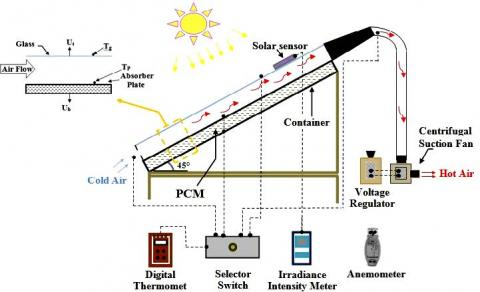Figure 2. Schematic diagram of the used system

Table 1. The paraffin wax thermo-physical properties

 Material Specification Cp (kJ/kg.K) k (W/m.K) ρ (kg/m3) Tm (°C) Г (kJ/kg) Paraffin wax Solid (2.0) Solid  (0.24) Solid  (910) 61.7 190 Liquid (2.15) Liquid (0.22) Liquid (790)
4. Results and Discussions

The tests were done in a clear sky day at (12,14 and 17 of march\2018) for the three cases of test, the selected test days is to close values of solar irradiance to give a clear Perception for the collector performance with the different cases of test, as shown in Figure 3. There is no large difference in irradiance values for these days. It was noted also, that the decreasing in absorber surface temperature with using PCM only about 12.6℃ at 12:30 PM, this decreasing in temperature was done by absorbing the heat energy from the absorber plate by PCM (paraffin wax) in charging heat process. While, the decreasing in absorber surface temperature with using PCM/Fins was about 16.6℃ at 12:30 PM, because the using of fins led to inject the temperature deeply in the paraffin wax and this led to more decreasing in the absorber temperature.

## 3.jpg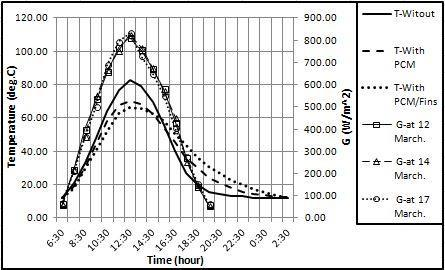Figure 3. Absorber surface temperatures and solar irradiance values with time in three days of test

At about 3:30 PM, the curves’ behavior are reversed in a discharging heat process, it seen that the absorber surface temperature with using PCM/Fins was higher than others and still heated a long time about 2 hours comparative with absorber without fins (PCM only), this behavior was due to the low thermal conductivity of the paraffin wax which led to keep the more heat energy that injected interior by the fins and rejected for a long time.

## 4.jpg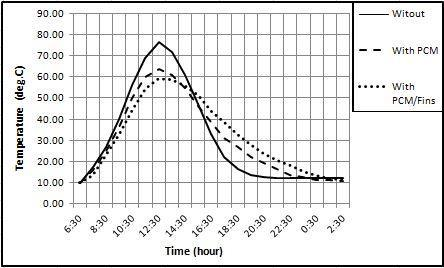Figure 4. Out let air temperature with time at (0.0038 kg/s) of air mass flow rate for the three test cases

## 5.jpg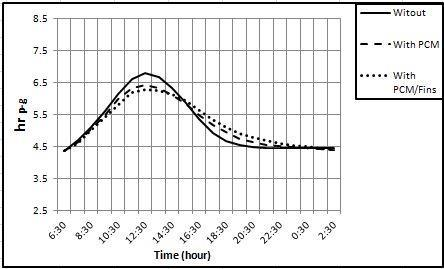Figure 5. Radiation heat transfer coefficients with time between the absorber plate and glass cover for the three test cases

## 6.jpg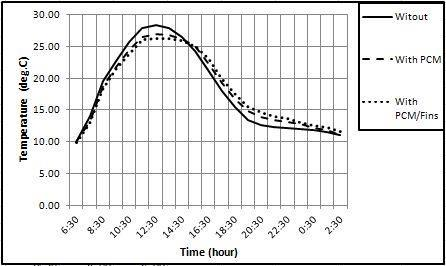Figure 6. The collectors glass cover temperature with time

Figure 4 gives the out let air temperature at 0.0038 kg/s of air mass flow rate for the three test cases. The radiation heat transfer coefficient between the absorber plate and glass cover are more effect on the collector glass cover temperature as shown in Figures 5 & 6 respectively, depending on Equation (13).

The convection heat transfer coefficient between absorber plate and collector glass cover for each case of test are more affected on Rayleigh number with time as shown in Figures 7 & 8, and it is depending on the absorber temperatures for each test case as displayed in Equations (9 and 10) respectively.

The values of convection heat transfer coefficient between absorber plate and collector glass cover are dominated on the Nusselt number values with time for each test case in as shown in Figure 9. The overall heat loss coefficients of the used collector are shown in Figure 10 depending on the experimental calculations by Equation (6).

## 7.jpg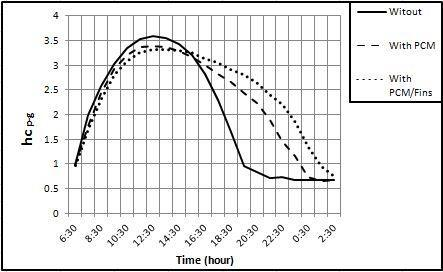Figure 7. The convection heat transfer coefficients with time between absorber plate and collector glass cover

## 8.jpg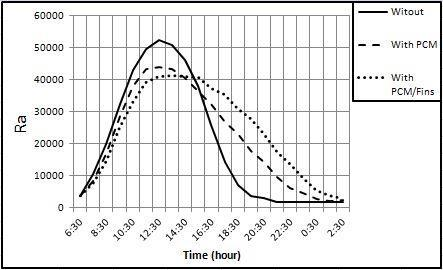Figure 8. Rayleigh number values with time for the three test cases

## 9.jpg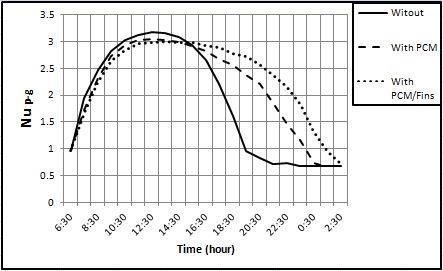Figure 9. Nusselt number values with time for the three test cases

## 10.jpg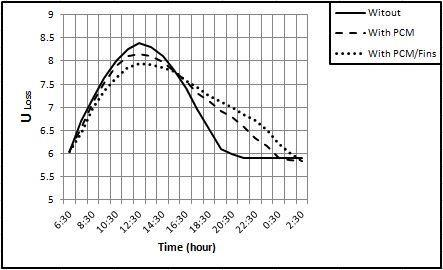Figure 10. Overall heat loss coefficients with time for the three test cases

Depending on Equations (18 and 19), the overall heat loss coefficients for each test cases affect on the energy and exergy losses from the collector as showed in Figure 11, for the two test cases of using the PCM (paraffin wax) only and PCM/Fins, the decreasing in the energy losses was about 24% and 33% respectively, comparative with the collector without PCM at the peak charge time (12:30 PM). While the decreasing in the exergy heat losses was about 30% and 40% respectively at the same time.

## 11.jpg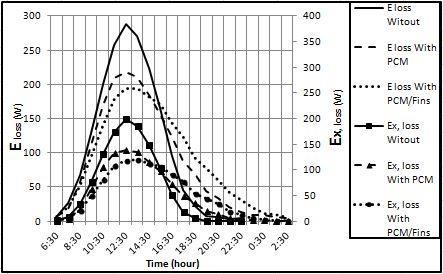Figure 11. Energy and exergy heat losses with time from the collector for the three test cases

While, Figure 12 shows the energy and exergy heat gains from the collector; the decreasing in the energy heat gain with using PCM was about 23% and about 32% with using PCM/Fins comparative with the collector without PCM at the peak charge time (12:30 PM). While, the decreasing in the exergy heat gain with using PCM only was about 34%, and about 45% with using PCM/Fins comparative with the collector without PCM at the same time. This decreasing in the energy and exergy heat gains was due to sum heat goes to store in the PCM for the two test cases.

## 12.jpg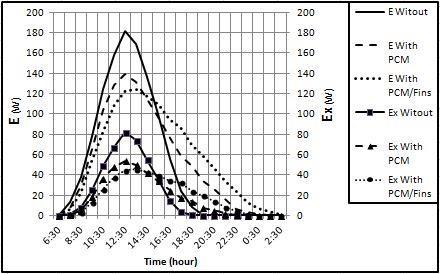Figure 12. Energy and exergy heat gains with time from the collector for the three test cases

## 13.jpgFigure 13. Energy and exergy efficiencies with time of the collector for the three test cases

## 14.jpg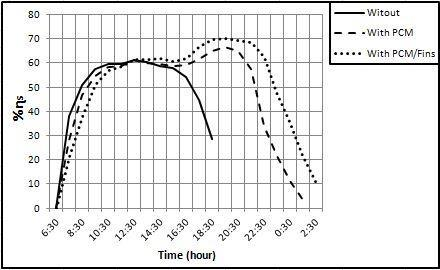Figure 14. Collectors thermal storage efficiency with time for the three test cases

The stored heat led to decreasing in both the energy and exergy of the collector efficiency as shown in Figure 13, the decreasing in the energy collector efficiency about 20% and 30% for the collectors with PCM and PCM/Fins respectively, comparative with the collector without PCM at the peak charge time, also, could be noted a decreasing in the exergy collector efficiency about 31% and 44% for the collectors with PCM and PCM/Fins respectively, comparative with the collector without PCM at the same time. The efficiency of the thermal storage could be concluded in Figure 14, at the discharge heat time (especially at 7:30 PM) could be note enhancing in the efficiency of the thermal storage of the collector with using longitudinal PCM/fins about 6% compared with collector used PCM only.

For the previous Figures (11-14), this behavior can be explained that the heat transferred during the charging process and the used fins play to injected the heat deeply into the wax and accelerated it melting during peak time, but during the discharge process the high thermal conductivity of the fins does not increase the heat transfer during discharge although it has a higher absorption surface, because of the heat remains stored internally in the wax layers away from the fins because of the low thermal conductivity of the wax, they move to the fins slower and this is the principle of the use of wax in a processes of thermal storage.

5. Conclusion

From the experimental results, it can conclude the following:

1. Using of PCM (paraffin wax) as a thermal storage heat material led to decreasing in the collector energy and exergy losses, the decreasing in the collector energy and exergy losses with using PCM/Fins are more than the collector with PCM only, comparative with the collector without PCM at the peak heat charging time (12:30 PM).
2. Due to sum heat goes to store in the PCM at heat charging time for the two cases with and without fins, the energy and exergy heat gains and efficiencies was decreased. This decrease is the most in the collector with using PCM/Fins comparative with the collector without PCM at the same test time.
3. Utilizing the longitudinal fins with PCM in the used solar air collector caused enhancing in the thermal storage efficiency comparative with the collector with PCM only, which could be noted at the time of the heat discharge.
Nomenclature
 A Area (m2). Cp Specific heat (kJ/kg.K). E Energy transferred by the collector (W). Ex Exergy transferred by the collector (W). g Constant of gravitational (9.81 m2/s). G Irradiation intensity (W/m2). hc Convective heat transfer coefficient (W/m2.k). hr Radiation heat transfer coefficient (W/m2.k). k Thermal conductivity(W/m.k). L Length (m). m' Mass fiow rate (kg/s). P Pressure (pa). Pr Prandtl number. R Specific gas constant for dry air (287.05 J/kg.K). Ra Rayleigh number. t Insulation thickness (m). T Temperature (oC). U Heat loss coefficient (W/m2.k). V Out let air velocity (m2). W Width (m). Greek letters $\mathcal{E}$ Emittance (0.88) for glass, (0.15) for absorber plate. $\eta$ Efficiency (%). $\rho$ Density (kg/m3). $\sigma$ $5.67 * 10^{-8}\left(\mathrm{W} / \mathrm{m}^{2}-\mathrm{K}^{4}\right)$ Г Latent heat of fusion (kJ/kg). v Air kinematic viscosity $\left(\mathrm{m}^{2} / \mathrm{s}\right)$ $\beta$ Collector tilt angle (deg). $\beta^{\prime}$ Expansion volumetric coefficient ( $\beta^{\prime}=1 / \mathrm{T}$ ). Subscripts a Air. atm Atmospheric. b Back side. b-a From the collector back side to ambient. c Collector. d Duct cross sectional area at outlet air. e Edge. g Glass. g-a From the collector glass cover to ambient. in Inlet. m Melting. out Outlet. p Absorber. p-g From the collector absorber plate to glass cover. s Storage. t Top.
References

 Akpinar, E.K., Koçyiğit, F. (2011). Energy and exergy analysis of a new flat-plate solar air heater having different obstacles on absorber plates. Applied Energy, 87(11): 3438-3450. https://doi.org/10.1016/j.apenergy.2010.05.017

 Abdelkader, T.K., Zhang, Y., Gaballah, E.S., Wang, S., Wan, Q., Fan, Q. (2019). Energy and exergy analysis of a flat-plate solar air heater coated with carbon nanotubes and cupric oxide nanoparticles embedded in black paint. Journal of Cleaner Production, 119501. https://doi.org/10.1016/j.jclepro.2019.119501

 Velmurugan, P., Kalaivanan, R. (2015). Energy and exergy analysis of solar air heaters with varied geometries. Arabian Journal for Science and Engineering, 40(4): 1173-1186. https://doi.org/10.1007/s13369-015-1612-2

 Esen, H. (2008). Experimental energy and exergy analysis of a double-ﬂow solar air heater having different obstacles on absorber plates. Building and Environment, 43: 1046-1054. https://doi.org/10.1016/j.buildenv.2007.02.016

 Chaabane, M., Mhiri, H., Bournot, P. (2014). Thermal performance of an integrated collector storage solar water heater (ICSSWH) with phase change materials (PCM). Energy Conversion and Management, 78: 897-903. https://doi.org/10.1016/j.enconman.2013.07.089

 Abdulmunem, A.R., Jalal, J.M. (2018). Indoor investigation and numerical analysis of PV cells temperature regulation using coupled PCM/Fins. International Journal of Heat and Technology, 36(4): 1212-1222. https://doi.org/10.18280/ijht.360408

 Lin, S.C. (2017). Numerical simulation of PCM integrated solar collector storage water heater. ARPN Journal of Engineering and Applied Sciences, 12(10): 3363-3367.

 Mehla, N., Yadav, A. (2017). Experimental analysis of thermal performance of evacuated tube solar air collector with phase change material for sunshine and off-sunshine hours. International Journal of Ambient Energy, 38(2): 130-145. https://doi.org/10.1080/01430750.2015.1074612

 Abdulmunem, A.R. (2017). Passive cooling by utilizing the combined pcm / aluminum foam matrix to improve solar panels performance: Indoor investigation. The Iraqi Journal for Mechanical and Material Engineering, 17(4): 712-723.

 Abed, A.H., Abdulmunem, A.R.  (2018). Investigation of combination between latent and sensible heat storage materials on the performance of flat plate solar air heater. The Iraqi Journal for Mechanical and Material Engineering, 18(1): 63-77. https://doi.org/10.32852/iqjfmme.Vol18.Iss1.73

 Abdulmunem, A.R., Abed, A.H., Qatta, H.I. (2016). Applicability of using thermal storage materials in solar air heater. The Iraqi Journal for Mechanical and Material Engineering, 16(1): 76-86.

 Aghbalou, F., Badia, F., Illa, J. (2006). Exergetic optimization of solar collector and thermal energy storage system. International Journal of Heat and Mass Transfer, 49: 1255-1263. https://doi.org/10.1016/j.ijheatmasstransfer.2005.10.014

 Duffie, J.A., Beckman, W.A. (2013). Solar Engineering of Thermal Processes. 4th Edition. John Wiley & Sons, New York, NY.

 Said, Z., Alim, M.A., Janajreh, I. (2015). Exergy efficiency analysis of a flat plate solar collector using graphene based nanofluid. IOP Conference Series: Materials Science and Engineering, 92: 012015.